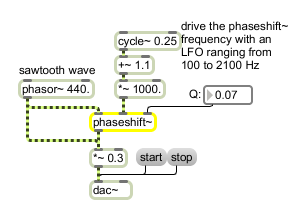# phaseshift~

Phase shifter

## Description

phaseshift~ is a 2nd-order phase shift filter. It provides a nonlinear delay time as a function of input frequency, emulating the phase shift elements found in analog phase shifter effects. Cascade several phaseshift~ objects to create a more dramatic effect. Since phase shifting by itself is inaudible in most circumstances, usually you'll want to mix the output of phaseshift~ with its input signal, so that frequency cancellations occur as the delayed signal is added to the original signal.

## Arguments

Name Type Opt Description
frequency and Q float opt If one argument is provided, it sets the phaseshift~ object's frequency parameter. If two arguments are provided, the first sets the frequency parameter and the second sets the Q factor.

## Messages

 int frequency/Q [int] An int or float can be sent in the middle or right inlets to change the frequency at which signals will be shifted by 180 degrees or the "Q" factor, respectively (see inlet descriptions above). If a signal is connected to one of the inlets, a number received in that inlet is ignored. float frequency/Q [float] An int or float can be sent in the middle or right inlets to change the frequency at which signals will be shifted by 180 degrees or the "Q" factor, respectively (see inlet descriptions above). If a signal is connected to one of the inlets, a number received in that inlet is ignored. list frequency and Q [list] A list of two numbers where the first number specifies the frequency parameter and the second number specifies the Q can be provided to set the object's parameters accordingly in any inlet. clear The word clear clears the object's sample-memory. signal In left inlet: the signal to be shifted in phase. In middle inlet: Sets the frequency at which signals will be shifted by 180 degrees. Signals below this frequency will be shifted less; signals above will be shifted more, up to 360 degrees. In right inlet: Sets the "Q" factor, or steepness with which the object's phase shift changes from zero to 360 degrees. Useful values for Q are generally in the range 1 to 10.

## Output

signal: The input signal, its the frequency components or harmonics shifted in phase from zero to 360 degrees, dependent upon their frequency and the values of the object's frequency and Q parameters.

## ExamplesSimulate an analog phase shifter using phaseshift~ and an LFO